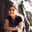a concise shot of dev knowledge
Become a Contributor
Concise shots of dev knowledge

RELATED TAGS

java
concurrency
communitycreator

# How to use the atomic compare and swap technique in JavaAnjana Shankar

### Compare and swap

Compare and swap (CAS) is a concurrent programming technique. It compares an expected value to the actual value of the variable and modifies it only if it matches.

### Compare and swap as an atomic operation

Modern CPUs have built-in support for atomic compare and swap operations.

Java5 onwards allows us to leverage the CPU functions via the set of classes in the java.util.concurrent.atomic package.

Some examples are:

• AtomicBoolean
• AtomicLong
• AtomicInteger

This is not an exhaustive list of classes in the Java atomic package.

Let’s see an example of how to use the AtomicInteger package.

### Example of AtomicInteger

import java.util.concurrent.atomic.AtomicInteger;

public class MyAtomicLong {
public static void main(String[] args) {
// Create an atomic integer
AtomicInteger atomicInteger = new AtomicInteger();

// Creating an atomic integer with an initial value
AtomicInteger atomicInteger123 = new AtomicInteger(123);

// Getting the atomic integer value
long theValue = atomicInteger123.get();

//Setting the value
atomicInteger123.set(234);

//Compare and set
int expectedValue = 123;
int newValue = 234;
atomicInteger123.compareAndSet(expectedValue, newValue);

}
}

Working with an AtomicInteger
• Line 6: We create an atomic integer without any initial value. In order to create an atomic integer with an initial value, we can use the syntax on line 9.

• Line 12: We get the value of an atomic integer.

• Line 15: We set a new value of the atomic integer.

• Line 20: We see the usage of the compareAndSet function. It first checks the value of the variable to see if it matches the expected value. It then goes ahead and sets the value to the new value. The function returns true if the value was set to the new value. If not, the function returns false.

• Line 24 and 25: We see how the getAndAdd and addAndGet methods are used. As the name suggests, the getAndAdd returns the value and then adds to it. Meanwhile, the addAndGet function first adds and then returns the resulting value.

### Conclusion

Because the set of atomic classes uses the CPU’s support for comparison and swap, these are faster than traditional locks.

RELATED TAGS

java
concurrency
communitycreator

CREATORAnjana Shankar
RELATED COURSES

View all Courses

Keep Exploring

Learn in-demand tech skills in half the time